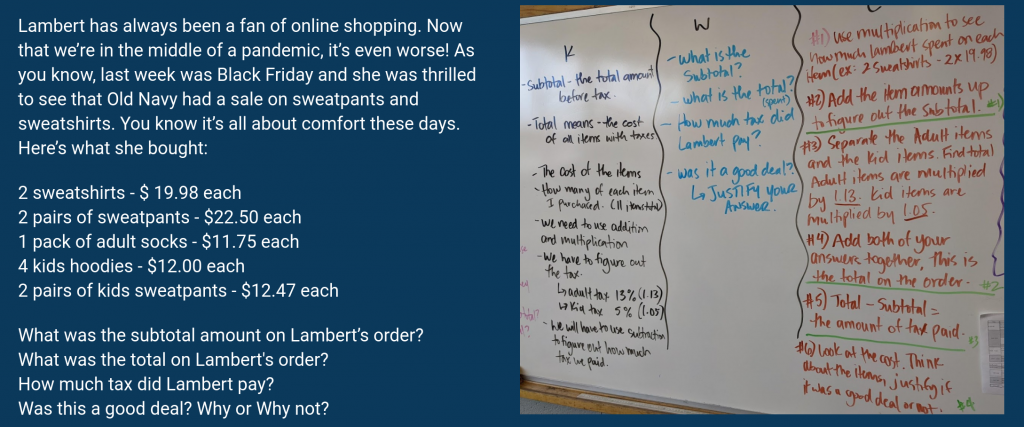Sadly, I can’t remember who introduced me to the KWC but it’s a strategy that I have used in Math with students for a number of years now and I should really find the person and thank them. If it was you, please reach out and let me know! Particularly when solving word problems, a KWC helps students to consider the question, what they know, what they want to know, and possible steps to take in getting started and solving the problem.

Prior to the break, we were working towards demonstrating the knowledge and skills needed to make informed financial decisions. Using problems that we would encounter in the real world, students were tasked with trying to solve them and to justify their choices. In this post, I break down the KWC using one of the guided examples in our classroom.

K – What do you know?

After reading the problem, students are asked to list everything that they know about the problem. These are things that they know for sure based on the information given and sometimes, their prior knowledge.

Here’s the question that we worked on as a class as we started learning about how to calculate the sales tax:As a class, we learned a new term – subtotal – and understood that total now meant the cost, including tax. We also determined that the operations we would use would be addition, multiplication, and subtraction. We learned that there were different rates for tax based on the type of item. In this case, adult clothing and children’s clothing.

W – What do you want to know?

This is the place where students can clearly state what they want to figure out, find out, or do. Restating the question in your own words helps to make sure that you really understand what is being asked in the question. In our case, this was a multi-step problem, so there were several things that we wanted to know:

• What is the subtotal?
• What is the total?
• How much tax was paid?
• Was it a good deal?

C – Conditions

This part is always one that is a little tricky for me. I often think of this section as the things that students need to watch out for but as in our case, I have used this section as the steps that we need to take in order to solve the problem. In other words, what are the things that we need to do to answer the question successfully? Through discussion, we broke down the problem and determined the steps involved in solving the problem so that when working in their groups, students could refer back to it. Even with the steps in place, there were groups of students who solved the problem differently. For example, for the third question, rather than taking the total and subtracting the subtotal to find the tax, some groups wanted to multiply the subtotal by the appropriate tax rate (0.13 or 0.05) and also state the amounts paid for the different rates. When it came to justifying their thinking for question 4, it was great to see that some groups asked questions about the discount for the sale. Many were eager to jump online to investigate the non-sale price and did calculations to determine how much of a deal it really was. Others thought about taking the total and dividing that by the number of items and thought about whether or not that was a deal based on the price per item. Different groups thought of different ways to use Math to justify their answer.

This question took us a couple of Math periods to work through but it continued to help solidify the effectiveness of the use of this strategy. With gradual release – beginning with the teacher as a guide; moving into students using it in small groups, then partners, and then on their own – once students understand the problems they come across, they are better able to solve them with greater confidence. While students aren’t always going to stop and work through creating their own KWC, it’s one tool that they can use and when given problems to solve. I’ve seen students use it independently and confidently to answer all parts of multi-step problems.

This site uses Akismet to reduce spam. Learn how your comment data is processed.